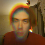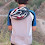## Monday, January 04, 2010

### Voting on Mathematical Truths: The Axiom of Det.

One of the founders of Conservapedia (a conservative alternative to Wikipedia) said the following on The Colbert Report:
There is an absolute truth. People don't vote on mathematical things like 2+2=4.
Given the source this quote may be ironic. However, this post is not about Conservapedia or Wikipedia. Its about voting on mathematical truths.

There is one kind of math where a vote might be appropriate. Some Set Theorists would like to resolve CH. We already know that this cannot be done in ZFC. So they want to add more axioms. What property should an axiom have? It should be obvious. It is unlikely that we will have new axioms of that type. How about that it be reasonable? Some set theorists think it is reasonable to remove FULL AC and add The Axiom of Determinacy (stated below). I want YOU to VOTE on if it is reasonable.

Definition: Let A be a subset of {0,1}&omega. Let GA be the following game: player I picks b1 &isin {0,1}, then player II picks b2 &isin {0,1}, then player I picks b3 &isin {0,1}, etc. If the final sequence b1 , b2 , b3 ... is in A then I wins. If not then II wins.

Definition: Let A be a subset of {0,1}&omega. A is determined if either player I or II has a winning strategy for GA.

The Axiom of Determinacy (AD): For all sets A that are subsets of {0,1}&omega, A is determined.
1. AD is known to be true for A a Borel Set (Donald Martin proofed that).
3. AD implies that every subset of the plane is measurable.
4. I don't think AD implies CH or not CH (are both AD + CH and AD + NOT(CH) known to have models?)
5. AD has a Wikipedia entry. This should not be taken as a sign that it is true of false. It should not even be taken as a sign that its well known. It just means someone put up an entry.
6. My wife thought AD was false in 1995 but true in 2005. I do not know what changed her mind. She is not a set theorist and had not thought about it in the intervening 10 years.
7. All finite games are determined so this is taking something true of finite games and assuming it is true for infinite games.
When you vote note that there is no right or wrong answer.

1.The axiom of choice is obviously true. The cartesian product of nonempty sets isn't just nonempty -- it is very large.

2.1) Thats a NO vote.

makes me at least question AC.

bill gasarch

3.4.My vote: It is not reasonable at all. I'm not a set theorist, and I don't see any reason why it should be true. A good argument could probably convince me, but then we must extract another, more reasonable axiom from that argument, and go with it instead of AD.

...I even have problems with the formalization of the Axiom, specifically, the definition of 'strategy'. Isn't it possible that the implications of the Axiom slightly depend on the exact formalization of the notion of strategy?

5.I'm very reluctant to call axioms true, and I consider both obviousness and reasonableness to be unsatisfying metrics to rate an axiom. I think the only measure should be the "interesting-ness" of the consequences (and of course consistency is of utmost importance).

I have three reasons for this view: 1, history is scattered with conclusions that weren't obvious (nor felt reasonable) until enough time passed to find more comprehensible descriptions; 2, there is no real need for mathematics to represent reality (and many interesting conclusions are the result of toy models that are very much not real); 3, the "interesting-ness" of the consequences of an axiom are the best motivators for researchers to research.

That said, I'm a physicist, and I only know enough CS and set theory to get myself in (uncountable) trouble.

Regardless, if voting is still important, I would vote that we have some people accept AC and others reject it, (based on their own personal intuitions about which is more interesting to study), which I believe is the status quo. So I am most likely no help at all.

6.Even among set theorists, most don't believe the axiom of choice is true. The idea is usually that AD should be true in L(R), not the actual universe. L(R) is the sets that are definable in some broad sense. So what this says is that any pathological sets - non meaurable, without the property of baire, etc., must be inherently very undefinable.

If certain large cardinals exist, than AD is actually true in L(R). Not just consistent, but actually true.

7.Anon4 again. I'd just like to note that I totally agree with cody's formalist position here, I just wanted to play along with GASARCH's platonist game.

8.Seems less intuitively reasonable to me than the axiom of choice.

Briefly: set theory and theory-of-computation and so on are minefields of stuff like the russell paradox, goedel-type incompleteness results, the halting problem, and so on.

Thus I get nervous taking as an axiom that, essentially, a particular class of questions always has a determined answer; I understand that the meaning of the AD is subtler than that but on such a short consideration I'm only going by analogy here.

By comparison the AC seems reasonable; if in the course of a proof you've shown that such-and-such set-of-sets exists it seems silly not to allow you to proceed to take out a representative member of each set...what issues I might have with the AC have more to do with which sets-of-sets it is or isn't "reasonable" to allow in a particular context, which I suspect is what really bothers people when they claim to be bothered by the AC.

(VIZ banach-tarski: is it really "reasonable" to unquestioningly accept that you "can" (in some sense) decompose S2 into uncountably many orbits, each of which is "dense" in S2? I accept banach-tarski as saying "if you could make that decomposition AND if you can then draw a representative point from each orbit THEN you can duplicate the sphere", but depending on which side of the bed i got up on that day sometimes my intuition balks much more at the notion of distinguishing the various dense orbits from each other than at subsequently choosing members from said orbits.)

9.I'm surprised people who read a computer science blog think the axiom of choice is obviously true. I would think that computer scientists prefer to work in a constructive topos - in other words, it's not the size of a Cartesian product that matters but whether any of it is accessible to a Turing machine!

10.Are we allowed to vote "Set theory is not a natural model for mathematics"?

The point being, a mathematical universe that is known to contain Conway's "Surreal Numbers" might perhaps contain even stranger (and possibly friendlier) mathematical beasts ...

Perhaps some person---who is very definitely not me---might say something sensible and/or provide a reference about surreal numbers, set theory, ZFC, and AC?

11.Axiom: Santa Claus is the objectification of the spirit of Christmas.

Therefore, Santa Claus is real.

12.@Qiaochu Yuan: Extending your reasoning about how you expect computer scientists to think, you would expect all computer scientists to be some brand of intuitionists. Yet, as someone who has been in both math and CS, I know very few true intuitionists, in either math or CS.

Most computer scientists with any mathematical bent learned their mathematics in the same undergrad (or sometimes grad) courses that mathematicians did, and (hence?) most have inherited the views that are either implicit or explicit in their teachers' teachings.

13.My quick intuition is that AD is false. Finite game trees have a unique value because one can start at the leaves and work upwards, evaluating each node as a min or max, but in an infinite tree where does one start?

I'm less sure about consequence 3, that every subset of the plane is measurable; considerations related to those for Freiling's axiom of symmetry suggest it might be true.

14.Although I like AD much better than AC, I would still vote for AC just because it is at least simpler to state and, to me, more intuitive. It's also a bit more axiom like than AD (maybe again due to its simpler statement).

15.The axiom uses the term "strategy" without definition. I'd naively think of a strategy as a recursive function from game states to moves, otherwise you couldn't carry it out. But you can't have that definition in mind, because then the axiom would be provably false (uncountably many games, but only countably many strategies). So what notion of "strategy" are you using?

16.Strategy is a function that,
given the game so far, outputs
a move. Winning strategy means
that if you use this to guide
17.18.